×#### Thank you for registering.

One of our academic counsellors will contact you within 1 working day.

Click to Chat

1800-1023-196

+91-120-4616500

CART 0

• 0

MY CART (5)

Use Coupon: CART20 and get 20% off on all online Study Material

ITEM
DETAILS
MRP
DISCOUNT
FINAL PRICE
Total Price: Rs.

There are no items in this cart.
Continue Shopping• Complete JEE Main/Advanced Course and Test Series
• OFFERED PRICE: Rs. 15,900
• View Details

```Chapter 16: Surface Area And Volume Exercise – 16.3

Question: 1

A bucket has top and bottom diameters of 40 cm and 20 cm respectively. Find the volume of the bucket if its depth is 12 cm. Also find the cost of tin sheet for making the bucket at the rate of Rs 1.20 per dm2

Solution:

Given Diameter to top of bucket = 40 cm

Radius (r1) = 40/2 = 20 cm

Diameter of bottom part of the bucket = 20 cm

Radius (r2) = 30/2 = 10cm

Depth of the bucket (h) = 12 cm= π/3(202 + 102 + 20 × 10)12

= 8800 cm3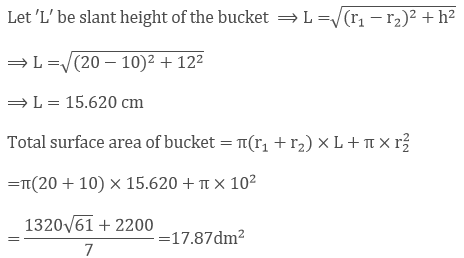Given that cost of tin sheet used for making bucket per dm2 = Rs 1.20

So total cost for 17.87dm2 = 1.20 × 17.87 = Rs 21.40. ?

Cost for 17.87dm2 = Rs 21.40

Question: 2

A frustum of a right circular cone has a diameter of base 20 cm, of top 12 cm and height 3 cm. find the area of its whole surface and volume.

Solution:

Given base diameter of cone (d1) = 20 cm

Radius (r1) = 20/2 cm = 10 cm

Top diameter of Cone (d2) = 12 cm

Radius (r2) = 12/2 cm = 6 cm

Height of the cone (h) = 3 cm

Volume of the frustum right circular cone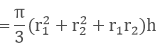= π/3(102 + 62 + 10 × 6)3

= 616 cm3

Let 'L' be the slant height of cone⟹ L = 5 cm

Slant height of cone (L) = 5 cm Total surface area of the cone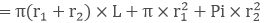= π(10 + 6) × 5 + π × 102 + Pi × 62

= π(80 + 100 + 36)

= π(216)

= 678.85 cm2

Total surface area of the cone = 678.85 cm2

Question: 3

The slant height of the frustum of a cone is 4 cm and perimeters of its circular ends are 18 cm and 6 cm. Find the curved surface of the frustum.

Solution:

Given slant height of frustum of cone (l) = 4 cm

Let ratio of the top and bottom circles be r1 and r2

Given perimeters of its ends as 18 cm and 6 cm

⟹ 2πr1 = 18 cm; 2πr2 = 6 cm

⟹ πr1= 9 cm ... (a)

πr2 = 3 cm ... (b)

Curved surface area of frustum cone = π(r1 + r2)l

= π(r1 + r2)l

= (πr1+πr2)l = (9 + 3) × 4 = (12) × 4 = 48 cm2

Curved surface area of the frustum cone = 48 cm

Question: 4

The perimeters of the ends of a frustum of a right circular cone are 44 cm and 33 cm. If the height of the frustum be 16 cm, find its volume, the slant surface and the total surface.

Solution:

Let the slant height of frustum right circular cone be LL = 16.1 cm

Slant height of the frustum cone = 20.37 cm

Curved surface area of the frustum cone = π(r1 + r2)l

= π(7 + 5.25)16.1

Curved surface area of the frustum cone = 619.65 cm3

Volume of the frustum cone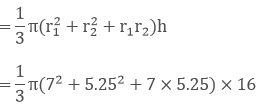= 1898.56 cm3

Volume of the cone = 1898.56 cm3

Total surface area of the frustum cone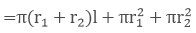= π(7 + 5.25) × 16.1 + π72 + π5.252

= π(7 + 5.25) × 16.1 + π(72 + 5.252) = 860.27 cm2

Total surface area of the frustum cone = 860.27 cm2

Question: 5

If the radius of circular ends of a conical bucket which is 45 cm high be 28 cm and 7 cm. Find the capacity of the bucket.

Solution:

Given Height of the conical bucket = 45 cm Radii of the 2 circular ends of the conical bucket is 28 cm and 7 cm r1 = 28 cm r2 = 7 cm

Volume of the conical bucket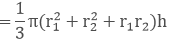= 1/3 π(282 + 72 + 28 × 7)45 = 15435π

Volume = 48510 cm3

Question: 6

If the radii of circular end of a bucket 24 cm high are 5 and 15 cm. Find surface area of the bucket.

Solution:

Given,

Height of the bucket (h) = 24 cm

Radius of the circular ends of the bucket 5 cm and 15 cm r1 = 5 cm; r2 = 15 cm

Let 'L' be the slant height of the bucket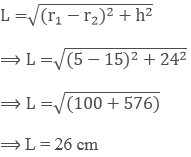Curved surface area of the bucket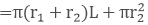= π(5 + 15)26 + π152 = π(520 + 225) = 745π cm2

Curved surface area of the bucket = 745π cm2

Question: 7

The radii of circular bases of a frustum of a right circular cone are 12 cm and 3 cm and the height is 15 cm. Find the total surface area and volume of frustum.

Solution:

Let slant height of the frustum cone be 'L' Given height of frustum cone = 12 cm

Radii of a frustum cone are 12 cm and 3 cm r1 = 12 cm; r2 = 3 cmL = 15 cm

Total surface area of cone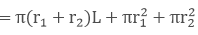= π(12 + 3)15 + π122 + π32

Total surface area = 378 cm2

Volume of frustum cone= 1/3 π(122 + 32 + 12 × 3) × 12 = 756π cm3
Volume of the frustum cone = 756 π cm3

Question: 8

A tent consists of a frustum of a cone capped by a cone. If radii of ends of frustum be 13 m and 7 m the height of frustum be 8 m and the slant height of the conical cap be 12 m. Find canvas required for the tent.

Solution:

Given height of frustum (h) = 8 m

Radii of the frustum cone are 13 cm and 7 cm

r1 = 13 m; r2 = 7

Let ‘L’ be slant height of the frustum cone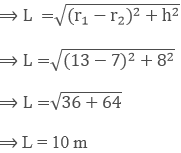Curved surface area of frustum (s1) = π(r1 + r2) × L= π(13 + 7) × 10 = 200 π m2

Curved surface area of frustum (s1)= 200 π m2

Given slant height of conical cap = 12 m

Base radius of upper cap cone = 7 m

Curved surface area of upper cap cone (s2) = πrL = π × 7 × 12 = 264 m2

Total canvas required for tent (S) = s1 + s2

S = 200π + 264 = 892.57m2

Total canvas = 892.57 m2

Question: 9

A bucket is in the form of a frustum of a cone with a capacity of 12308.8 cm3 of water. Radii of the top and bottom circular ends are 20 cm and 12 cm respectively. Find the height of the bucket and the area of the metal sheet used in its making.

Solution:

Radii of top circular ends (r1) = 20 cm

Radii of bottom circular end of bucket (r2) = 12 cm

Let height of bucket be ‘h’ Volume of frustum cone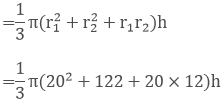= 784/3 πh cm3 ... (a)

Given capacity/ volume of the bucket = 12308.8 cm3 ... (b) Equating (a) and (b)

⟹ 784/3 πh cm3 = 12308.8⟹ h =15 cm

Height of the bucket (h) = 15 cm

Let 'L' be slant height of bucket

⟹ L2 = (r1 − r2)2 + h2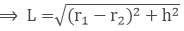⟹ L = 17 cm

Length of the bucket/slant height of the bucket (L) = 17 cm

Curved surface area of bucket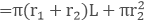= π(20 + 12)17 + π × 122

= π(9248 + 144) = 2160.32 cm²

curved surface area = 2160.32 cm²

Question: 10

A bucket made of aluminum sheet is of height 20 cm and its upper and lower ends are of radius 25 cm and 10 cm.  Find cost of making if the aluminum sheet costs Rs 70 per 100 cm2.

Solution:

Given height of bucket (h) = 20 cm

Upper radius of bucket (r1) = 25 cm

Lower radius of the bucket (r2) = 10 cm

Let 'L' be slant height of the bucket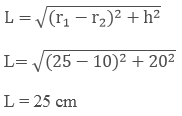Slant height of bucket (L) = 25 cm

Curved surface area of bucket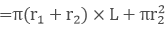= π(25 + 10) × 25 + π × 102 = 3061.5 cm2 ?

Curved surface area = 3061.5 cm2

Cost of making bucket per 100 cm2 = Rs 70

Cost of making bucket per 3061.5 cm2 = 3061.5/100 × 70 = Rs 2143.05

Total cost for 3061.5 cm2 = Rs 2143.05

Question: 11

Radii of circular ends of a solid frustum of a cone are 33 cm and 27 cm and its slant height is 10 cm. Finds its total surface area.

Solution:

Given slant height of frustum cone = 10 cm

Radii of circular ends of frustum cone are 33 cm and 27 cm r1 = 33 cm; r2 = 27cm

Total surface area of a solid frustum of cone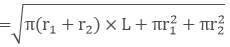= π(33 + 27) × 10 + π332 + π272

= π(60) × 10 + π332 + π272

= π(600 + 1089 + 729)

= 2418π cm2

Total surface area = 7599.42 cm2

Question: 12

A bucket made up of a metal sheet is in from of a frustum of cone of height 16 cm with diameters of its lower and upper ends as 16 cm and 40 cm. Find the volume of the bucket. Also find the cost of the bucket if the cost of metal sheet used id Rs 20 per 100 cm2.

Solution:

Given height of frustum cone = 16 cm

Diameter of the lower end of the bucket d1 = 16 cm

Lower end radius r1 = 16/2 = 8 cm

Upper end radius r2 = 340/2 = 20 cm

Let 'L' be slant height of frustum of cone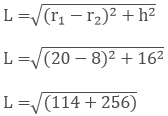L = 20 cm

Slant height of the frustum cone L = 20 cm

Volume of the frustum cone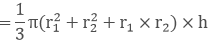=1/3π(82 + 202 + 8 × 20) × 16

Volume of the frustum cone = 10449.92 cm3

Curved surface area of the frustum cone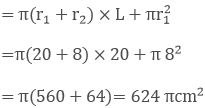Cost of the metal sheet per 100 cm2 = Rs 20

Cost of the metal sheet per 624 π cm2 = 624π/100 × 20

Cost of the metal sheet = 391.9

Question: 13

A solid is in the shape of a frustum of a cone. The diameters of two circular ends are 60 cm and 36 cm and height is 9 cm. find area of its whole surface and volume.

Solution:

Given height of the frustum cone = 9 cm

Lower end radius r1 = 60/2 = 30cm

Upper end radius r2 = 36/2 = 18 cm

Let slant height of the frustum cone be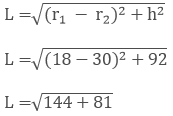L = 15 cm

Volume of the frustum cone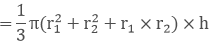=1/3π(302 + 182 + 30 × 18) × 9 = 5292π cm3

Volume = 5292π cm3

Total surface area of frustum cone= π(30 + 18) × 15 + π302 + π182

= π(720 + 900 + 324)

= 1944 Picm2

Total surface area = 1944π cm2

Question: 14

A milk container is made of metal sheet in the shape of frustum cone whose volume is 10459 cm3. The radii of its lower and upper circular ends are 8 cm and 20 cm. Find the cost of metal sheet used in making container at a rate of Rs 1.40 per cm2.

Solution:

Given,

Lower end radius r1 = 8 cm

Upper end radius r2 = 20 cm

Let the height of the container be 'h'

V1 = 1/3π (82 + 202 + 8(20))h cm3   .... (1)

Volume of the milk container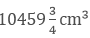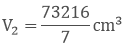V1 - V2

1/3π(82 + 202 + 8(20))h cm3⟹ h = 16 cm

Height of frustum cone (h) = 16 cm

Let slant height of frustum cone be 'L'L = 20 cm

Slant height of frustum cone (L) = 20 cm

Total surface area of the frustum cone= π(20 + 8 × 20 + π202 + π82

= π(560 + 400 + 64)

= 1024π

= 3216.99 cm2

Total surface area of the frustum = 3216.99 cm2

Question: 15

A reservoir in form of frustum of a right circular cone contains 44 × 107 liters of water which fills it completely. The radii of bottom and top of the reservoir are 50 m and 100 m. Find the depth of water and lateral surface area of the reservoir.

Solution:

Let depth of frustum cone be h Volume of first coner1 = 50 m; r2 = 100Volume of the reservoir = 44 × 107 liters .... (b)

Equating (a) and (b) 1/3 π(8500)h = 44 × 107 h = 24

Question: 16

A metallic right circular cone 20 cm high and whose vertical angle is 90° is cut into two parts at the middle point of its axis by a plane parallel to the base. If frustum so obtained be drawn into a wire of diameter 1/16 cm. Find the length of the wire.

Solution:

Let ABC be the cone.

Height of metallic cone AO = 20 cm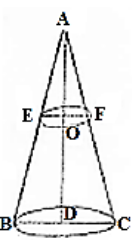Cone is cut into two parts at the middle point of its axis

Hence height of frustum cone AD = 10 cm

Since angle A is right angles.

So each angles B and C = 45°

Angles E and F = 45°

Let radii of top and bottom circle of frustum

Cone be r1 and r2

⟹ r1/10 = 1 ⟹ r1 = 10 cm

From ΔAOB

⟹ OE/OA = Cot 45°

⟹ r2/20 = 1

⟹ r2 = 20 cm
```### Course Features

• 728 Video Lectures
• Revision Notes
• Previous Year Papers
• Mind Map
• Study Planner
• NCERT Solutions
• Discussion Forum
• Test paper with Video Solution